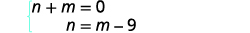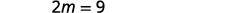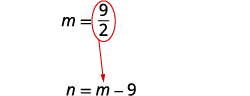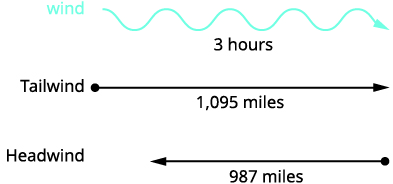# 4.3：使用方程组求解应用程序

•• OpenStax
• OpenStax
$$\newcommand{\vecs}{\overset { \rightharpoonup} {\mathbf{#1}} }$$ $$\newcommand{\vecd}{\overset{-\!-\!\rightharpoonup}{\vphantom{a}\smash {#1}}}$$$$\newcommand{\id}{\mathrm{id}}$$ $$\newcommand{\Span}{\mathrm{span}}$$ $$\newcommand{\kernel}{\mathrm{null}\,}$$ $$\newcommand{\range}{\mathrm{range}\,}$$ $$\newcommand{\RealPart}{\mathrm{Re}}$$ $$\newcommand{\ImaginaryPart}{\mathrm{Im}}$$ $$\newcommand{\Argument}{\mathrm{Arg}}$$ $$\newcommand{\norm}{\| #1 \|}$$ $$\newcommand{\inner}{\langle #1, #2 \rangle}$$ $$\newcommand{\Span}{\mathrm{span}}$$ $$\newcommand{\id}{\mathrm{id}}$$ $$\newcommand{\Span}{\mathrm{span}}$$ $$\newcommand{\kernel}{\mathrm{null}\,}$$ $$\newcommand{\range}{\mathrm{range}\,}$$ $$\newcommand{\RealPart}{\mathrm{Re}}$$ $$\newcommand{\ImaginaryPart}{\mathrm{Im}}$$ $$\newcommand{\Argument}{\mathrm{Arg}}$$ $$\newcommand{\norm}{\| #1 \|}$$ $$\newcommand{\inner}{\langle #1, #2 \rangle}$$ $$\newcommand{\Span}{\mathrm{span}}$$$$\newcommand{\AA}{\unicode[.8,0]{x212B}}$$

##### 学习目标

• 解决直接翻译应用程序
• 求解几何应用程序
• 解决均匀运动应用程序

1. 两个数字和九的总和为 31。 找到号码。
如果你错过了这个问题，请查看 [链接]
2. 双胞胎乔恩和罗恩去年共赚了96,000美元。 罗恩赚了8000美元，是乔恩收入的三倍。 每对双胞胎赚了多少钱？
如果你错过了这个问题，请查看 [链接]
3. 特快列车和当地火车离开匹兹堡前往华盛顿特区。特快列车可以在四个小时内出行，而当地火车则需要五个小时才能到达。 特快列车的速度比当地列车的速度快12英里/小时。 找出两列火车的速度。
如果你错过了这个问题，请查看 [链接]

## 解决直接翻译应用程序

##### 使用方程组求解应用程序。
1. 阅读问题。 确保所有文字和想法都被理解。
2. 确定我们在寻找什么。
3. 出我们要找的东西。 选择变量来表示这些数量。
4. 转换为方程组。
5. 使用良好的代数技巧求解方程组。
6. 检查问题中的答案并确保答案合理。
7. 用完整的句子@@ 回答问题。

 第 1 步。 阅读问题。 第 2 步。 确定我们在寻找什么。 我们正在寻找两个数字。 第 3 步。 说出我们要找的东西。 让$$n= \text{the first number}$$。 $$m= \text{the second number}$$ 第 4 步。 转换为方程组。 两个数字的总和为零。一个数字比另一个数字少九个。系统是：第 5 步。 求解 方程组。 我们将使用替换， 因为第二个方程为 n 求解。 在第一个方程中用 m − 9 代替 n。求解 m。代$$m=\frac{9}{2}$$入第二个方程， 然后求解 n。第 6 步。 检查问题中的答案。 这些数字在问题中有 意义吗？ 我们会把这个留给 你！ 第 7 步。 回答问题。 数字是$$\frac{9}{2}$$和$$−\frac{9}{2}$$。
##### 示例$$\PageIndex{2}$$

$$3, 7$$

##### 示例$$\PageIndex{3}$$

$$2, −8$$

 第 1 步。 阅读问题。 第 2 步。 确定我们在寻找什么。 我们正在寻找能够使薪酬相等 的 培训课程数量。 第 3 步。 说出我们要找的东西。 让 s=s= 希瑟的薪水。 n=n= 训练课程的次数 第 4 步。 转换为方程组。 选项 A 将向她支付 25,000 美元， 另加每次 训练的 15 美元。选项 B 将为每次培训课程向她支付 10,000 美元 + 40 美元。系统如图所示。第 5 步。 求解方程组。 我们将使用替代。在第二个 方程中用 25,000 +15 n 代替 s。求解 n。第 6 步。 检查答案。 每年 600 次培训课程是否合理？ 当 n = 600 时，这两个选项是否相等？ 第 7 步。 回答问题。 600 次培训课程的薪金选项将相同。

160 个策略

##### 示例$$\PageIndex{6}$$

Kenneth目前以22,000美元的薪水为A公司出售西装，每售出一套西装可获得10美元的佣金。 B公司为他提供薪水为28,000美元的职位，每售出一套西装可获得4美元的佣金。 Kenneth 需要卖出多少套西装才能使期权相等？

1000 套西装

##### 示例$$\PageIndex{7}$$

 第 1 步。 阅读问题。 第 2 步。 确定我们在寻找什么。 我们正在寻找 椭圆训练器每分钟 消耗的卡路里数量，以及每分钟循环训练消耗的卡 路里数量。 第 3 步。 说出我们要找的东西。 让 e=e= 椭圆训练机每 分钟消耗的卡路里数。 c=c= 循环训练时每 分钟消耗的卡路里数 第 4 步。 转换为方程组。 在椭圆机上 10 分钟和循环 训练 20 分钟，消耗 278 卡路里在椭圆机上 20 分钟和 30 分钟的循环训练消耗了 473 卡路里的热量系统是：第 5 步。 求解方程组。 将第一个方程乘以 −2 得到 e 的 相反系数。简化并添加方程。 求解 c。将 c = 8.3 替换为 原始方程之一来求解 e。第 6 步。 检查问题中的答案。 自己检查数学。第 7 步。 回答问题。 Jenna 在使用椭圆机训练时每分钟 消耗 8.3 卡路里的热量，每 分钟消耗 11.2 卡路里的热量。
##### 示例$$\PageIndex{8}$$

Mark 去健身房做了 40 分钟的 Bikram 热瑜伽和 10 分钟的跳千斤顶。 他消耗了 510 卡路里的热量。 下次他去健身房时，他做了 30 分钟的 Bikram 热瑜伽和 20 分钟的跳千斤顶，消耗 470 卡路里的热量。 瑜伽每分钟消耗多少卡路里？ 每跳千斤顶消耗多少卡路里？

Mark 每分钟瑜伽消耗 11 卡路里的热量，每分钟跳千斤顶消耗 7 卡路里的热量。

## 求解几何应用程序

##### 示例$$\PageIndex{10}$$

$$\begin{array} {ll} {\textbf{Step 1. Read }\text{the problem.}} &{} \\ {\textbf{Step 2. Identify }\text{what we are looking for.}} &{\text{We are looking for the measure of each}} \\ {} &{\text{angle.}} \\ {\textbf{Step 3. Name }\text{what we are looking for.}} &{\text{Let} x=\text{ the measure of the first angle.}} \\ {} &{\hspace{3mm} y= \text{ the measure of the second angle}} \\ {\textbf{Step 4. Translate }\text{into a system of}} &{\text{The angles are complementary.}} \\ {\text{equations.}} &{\hspace{15mm} x+y=90} \\ {} &{\text{The difference of the two angles is 26}} \\ {} &{\text{degrees.}} \\ {} &{\hspace{15mm} x−y=26} \\ {} &{} \\ {} &{} \\ {\text{The system is shown.}} &{\hspace{15mm} \left\{ \begin{array} {l} x+y=90 \\ x−y=26 \end{array} \right. } \\ {} &{} \\ {} &{} \\ {\textbf{Step 5. Solve }\text{the system of equations} } &{\hspace{15mm} \left\{ \begin{array} {l} x+y=90 \\ \underline{x−y=26} \end{array} \right. } \\ {\text{by elimination.}} &{\hspace{21mm} 2x\hspace{4mm}=116} \\ {} &{\hspace{28mm} x=58} \\ {} &{} \\ {} &{} \\ {\text{Substitute }x=58\text{ into the first equation.}} &{\hspace{15mm} x+y=90} \\ {} &{\hspace{14mm} 58+y=90} \\ {} &{\hspace{22mm} y=32} \\ {\textbf{Step 6. Check }\text{the answer in the problem.}} &{} \\ {} &{} \\ {} &{} \\ {} &{} \\ {\hspace{15mm} 58+32=90\checkmark} &{} \\ {\hspace{15mm} 58−32=26\checkmark} &{} \\ {\textbf{Step 7. Answer }\text{the question.}} &{\text{The angle measures are 58 and 32 degrees.}} \end{array}$$

##### 示例$$\PageIndex{13}$$

 第 1 步。 阅读问题。 第 2 步。 确定我们在寻找什么。 我们正在寻找每个 角度的测量方法。 第 3 步。 说出我们要找的东西。 假设 x=x= 第一个角度的度量。 y=y= 第二个角度的度量 第 4 步。 转换为方程组。 角度是补充性的。较大的角度为十二小于较小角度的五 倍。系统如下所示： 步骤 5。 求解方程替换系统。在第一个方程中用 5 x − 12 代替 y。 求解 x。在第二个 方程中用 32 代替 x，然后求解 y。第 6 步。 检查问题中的答案。第 7 步。 回答问题。 角度测量值分别为 148 和 32 度。

##### 示例$$\PageIndex{16}$$

 第 1 步。 阅读问题。第 2 步。 确定你在找什么。 我们正在寻找角度的测量方法。 第 3 步。 说出我们要找的东西。 假设 a=a= 第一个角度的度量。 b=b= 第二个角度的度量 第 4 步。 转换为方程组。 直角三角形中一个小角度的测量值是另一个小角度的三倍以上的十倍。三角形角度的测量总和为 180。系统如图所示。第 5 步。 求解方程组。 我们将使用替换，因为第一个方程已求解 a。在第二个方程中，用 3b+103b+10 代替 a。求解 b。将 b=20b=20 替换到第一个方程中，然后求解 a。第 6 步。 检查问题中的答案。 我们会把这个留给你！ 第 7 步。 回答问题。 小角度的测量值为 20 和 70 度。
##### 示例$$\PageIndex{17}$$

$$22, 68$$

##### 示例$$\PageIndex{18}$$

$$36, 54$$

##### 示例$$\PageIndex{19}$$

 第 1 步。 阅读问题。 第 2 步。 确定你在找什么。 我们正在寻找长度和宽度。第 3 步。 说出我们要找的东西。 假设 L=L = 围栏码的长度。 W=W= 围栏院子的宽度 第 4 步。 转换为方程组。 一个长度和两个宽度等于 125。长度将超过宽度的 四倍 5 英尺。系统如图所示。 第 5 步。 通过替换求解方程组。用 L = 4 W + 5 代入第一个 方程，然后求解 W。在第二个 方程中用 20 代替 W，然后求解 L。第 6 步。 检查 问题中的答案。第 7 步。 回答方程式。 长度为 85 英尺，宽度为 20 英尺。

## 解决均匀运动应用程序

##### 示例$$\PageIndex{22}$$$$\hspace{85mm} 65j=78k \nonumber$$

$$\hspace{105mm} k=j-\frac{1}{2} \nonumber$$

$$\begin{array} {ll} {\text{Now we have the system.}} &{\left\{ \begin{array} {l} k=j−\frac{1}{2} \\ 65j=78k \end{array} \right.} \\ {\textbf{Solve }\text{the system of equations by substitution.}} &{} \\ {} &{} \\ {\text{Substitute }k=j−12\text{ into the second equation,}} &{} \\ {\text{then solve for }j.} &{} \\ {} &{65j=78k} \\ {} &{65j=78(j−\frac{1}{2})} \\ {} &{65j=78j−39} \\ {} &{−13j=−39} \\ {} &{j=3} \\{\begin{array} {l} {\text{To find Kelly’s time, substitute }j=3 \text{ into the first}} \\ {\text{equation, then solve for }k.} \end{array} } &{k=j−\frac{1}{2}} \\ {} &{k=3−\frac{1}{2} } \\ {} &{k=\frac{5}{2} \text{ or } k=2\frac{1}{2}} \\ {\textbf{Check }\text{the answer in the problem.}} &{} \\ {\begin{array} {lllll} {\text{Joni}} &{3 \text{ hours}} &{(65\text{ mph})} &= &{195\text{ miles}} \\ {\text{Kelly}} &{2\frac{1}{2} \text{ hours}} &{(78\text{ mph})} &= &{195\text{ miles}} \end{array}} &{} \\ {\text{Yes, they will have traveled the same distance}} &{} \\{\text{when they meet.}} &{} \\ {\textbf{Answer }\text{the question.}} &{} \\ {} &{\text{Kelly will catch up to Joni in}} \\ {} &{2\frac{1}{2}\text{ hours. By then, Joni will}} \\ {} &{\text{have traveled }3 \text{ hours.}} \\ \end{array}$$

##### 示例$$\PageIndex{24}$$##### 示例$$\PageIndex{25}$$

 阅读问题。 这是一个均匀的运动问题，一 张照片可以帮助我们直观地看到情况。确定我们在寻找什么。 我们正在寻找飞船在静止水 中的速度和潮流的速度。 说出我们要找的东西。 让$$s= \text{the rate of the ship in still water.}$$ $$c= \text{the rate of the current}$$ 图表将帮助我们整理信息。 这艘船先下游再往上游。 向下游，潮流对 船舶有帮助，因此该船的实际费率为 s + c。 向上游移动，潮流会减慢飞船 的速度，因此实际速率为 s − c。下游需要 4 个小时。 上游需要 5 个小时。 单程距离为 60 英里。 转换为方程组。 由于速率时间就是距离，我们可以 编写方程组。求解方程组。 Distribute 使两个方程都 采用标准形式，然后通过消法求解。将顶部方程乘以 5，将 底部方程乘以 4。 添加方程，然后求解 s。将 s = 13.5 替换为原始方 程式。检查问题中的答案。 下游速度为 $$13.5+1.5=15$$英里每小时. 在 4 小时内船将行驶 $$15·4=60$$数英里。 上游速度为 $$13.5−1.5=12$$英里每小时. 在 5 小时内，船将行驶 $$12·5=60$$数英里。 回答问题。 飞船的速度为13.5英里/小时 ，目前的速度为1.5英里/小时。

##### 示例$$\PageIndex{28}$$

 阅读问题。 这是一个均匀的运动问题，一 张图片可以帮助我们进行可视化。确定我们在寻找什么。 我们正在寻找喷气式飞机 在静止空气中的速度和风速。 说出我们要找的东西。 让 j=j= 喷气机在静止空气中的速度。 w=w= 风速。 图表将帮助我们整理信息。 喷气式飞机有两次飞行——一次是顺风 ，一次是逆风。 在顺风中，风有助于喷气式飞机，因此 速率为 j + w。 在逆风中，风会减慢喷气机的速度， 因此速率为 j − w。每次旅行需要 3 个小时。 飞机顺风飞行 1,095 英里。 在逆风中，喷气式飞机飞行了987英里。 转换为方程组。 由于速率时间就是距离，所以我们得到方 程组。求解方程组。 分发，然后通过消除求解。 相加，然后求解 j。将 j = 347 替换为原始方 程之一，然后求解 w。检查问题中的答案。 顺风, 喷气式飞机的实际速度将为 $$347+18=365$$ mph. 在 3 小时内，喷气式飞机将行驶数 $$365·3=1,095$$英里 进入逆风，喷气式飞机的实际 速度将为 $$347−18=329$$英里每小时。 飞机将在3小时内行驶 $$329·3=987$$数英里。 回答问题。 喷气式飞机的速度为 347 英里/小时，风 速为 18 英里/小时。

• 方程组

## 关键概念

• 如何使用方程组求解应用程序
1. 阅读问题。 确保所有文字和想法都被理解。
2. 确定我们在寻找什么。
3. 出我们要找的东西。 选择变量来表示这些数量。
4. 转换为方程组。
5. 使用良好的代数技巧求解方程组。
6. 检查问题中的答案并确保答案合理。
7. 用完整的句子@@ 回答问题。